## Finding Unknown Angles

Practice Unlimited Questions

#### ANGLES

This lesson covers the following:

#### 1. Finding the sum of angles on a straight line

Example 1:
Consider a straight line ABC. Draw a line DB on to it meeting it at B.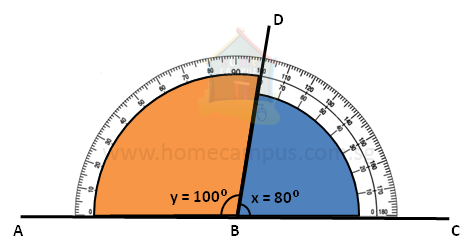Now, using a protractor, measure ∠x and ∠y.
In the figure above,
∠x  =  80°
∠y  =  100°
∠x  +  ∠y  =  80°  +  100°  =  180°
Example 2:
Consider the straight line ABC below. Drop a few lines onto point B and, using a protractor, measure all the angles formed.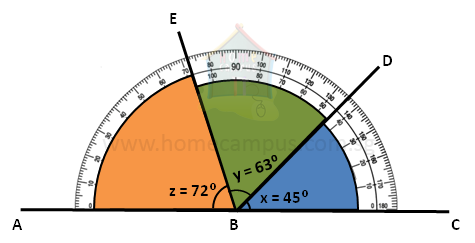In the figure above, we have dropped 2 lines DB and EB, and the angles formed by them are as follows:
∠x  =  45°
∠y  = 63°
∠z  = 72°
∠x  +  ∠y    +  ∠z  =  45°  +  63°  +  72°  =  180°

From the examples above it is clear that the sum of angles on a straight line is 180°.

#### 2. Finding the sum of angles at a point

Example 1:
Consider 2 straight lines AB and CD crossing at point O.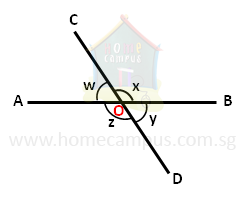We know that,
∠w + ∠x = 180° (sum of angles on a straight line is 180°)

Similarly,
∠y + ∠z is 180° (sum of angles on a straight line is 180°)

Adding all the angles at O, we get,
∠w + ∠x + ∠y + ∠z = 180° + 180° = 360°

Example 2:
Mark a point O and extend a few lines in any direction from it as below.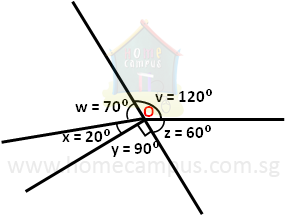Now using a protractor measure all the angles at point O. In our case,
∠v  =  120°
∠w  = 70°
∠x  = 20°
∠y  = 90°
∠z  = 60°
∠v  +  ∠w    +  ∠x  +  ∠y    +  ∠z  =  120°  +  70°  +  20°  +  90°  +  60°  =  360°

From the examples above it is clear that the sum of angles at a point is 360°.

#### 3. Vertically Opposite Angles

Consider 2 straight lines AB and CD crossing each other.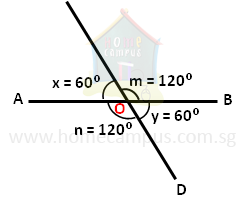Now, using a protractor measure ∠m, ∠x, ∠n and ∠y.
In our case,
∠m  =  120°
∠x  = 60°
∠n  = 120°
∠y  = 60°
∠m and ∠n form one pair of vertically opposite angles.

∠x and ∠y form a second pair of vertically opposite angles.
What we notice is,
∠m  =  ∠n ,   and
∠x  =  ∠y

Therefore, vertically opposite angles are equal to each other.

#### 4. CHALLENGE

Following are 3 straight lines crossing each other at O.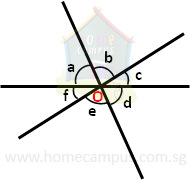Can you mark the three pairs of vertically opposite angles?
[Help: You may draw similar lines on a piece of paper and use a protractor to measure the angles.]